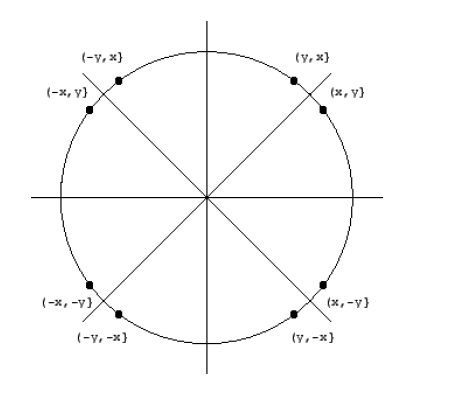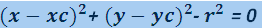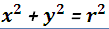## Computer Graphics and its Terminology

Computer Graphics:- Computer graphics are graphics created using computers and the representation of image data by a computer specifically with help from specialized graphic hardware and software.

Output Primitives:- Graphic SW and HW provide subroutines to
describe a scene in terms of basic geometric structures called output primitives.Output primitives are combined to form complex structures.

Simplest primitives are
>Point (pixel)
>Line segment.

Scan conversion:- The process of representing continuous graphics object as a collection of discrete pixels is called Scan Conversion.

Scan converting a Line :-  Scan converting a line means to draw pixels on an integer coordinate system in such a way that these pixels are as close to the actual line as possible. To draw a line we need two points, the starting point (x1,y1) of the line and the ending point (x2,y2). x1, y1,x2,y2 represents the coordinates of the points. Mathematically a line can be represented by the following slope intercept form:
y = m x + c

This equation is used to find any point on the line and is true for all the points on the line. Here
x is the x coordinate of the point.
y is the y coordinate of the point.
m is the slope of the line.
c is the y intercept.

Scan converting a Circle :-  A circle is a geometric figure which is round, and can be divided into 360 degrees. A circle is a symmetrical figure which follows 8-way symmetry.

8-Way symmetry: Any circle follows 8-way symmetry. This means that for every point (x,y) 8 points can be plotted. These (x,y), (y,x), (-y,x), (-x,y), (-x,-y), (-y,-x), (y,-x), (x,-y).For any point (x+a, y+b), points (x ± a, y ± b) and (y ± a, x ± b) also lie on the same circle. So it is sufficient to compute only 1/8 of a circle, and all the other points can be computed from it.

Drawing a circle: To draw a circle we need two things, the coordinates of the centre and the radius of the circle.

Radius: The radius of a circle is the length of the line from the centre to any point on its edge.

Equation of the circle: For any point on the circle (x,y) and the centre at point (xc,yc), the equation of the circle isHere r is the radius of the circle. If the circle has origin (0,0) as its centre then the above equation can be reduced to2D-Transformation :- Transformation is the fundamental part of computer graphics.Transformation means repositioning any geomatric object to another location by translation,rotation,scaling or by by any other transformation technique.

There are five types of transformation in computer graphics –
>Translation
>Rotation
>Scaling
>Reflection
>Shearing

Translation :-  In Translation an object is displaced to a given distance and direction from its original position.

Rotation :-  In Rotation an object is displaced by an angle θ in anticlockwise direction along x-axis w.r.t. origin.

Scaling :- Scaling is the process of expanding or compressing the dimensions of an object.

Reflection :-  Reflection is defined as the mirror image/symmetry of a point w.r.t axis.

Shearing :-  Shearing is defined as tilting in a given distance.Shearing transformation distort the shape of an object.

3D-Transformation :- 3D-Transformation is similar to 2D Transformations, which used 3x3 matrices. 3D transformations use 4X4 matrices (X, Y, Z, W)

Window to viewport mapping :- Window-to-Viewport mapping is the process of mapping or transforming a two-dimensional, world-coordinate scene to device coordinates.

Clipping :- Clipping is the process of cutting that part of the image which is to be displayed on the view port.
It includes all the procedures tha identify whether a portion of a graphic object is within inside or outside to a specified region.This region is called clliping window.